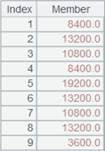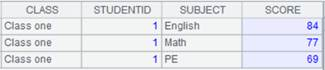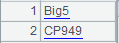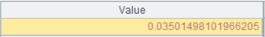# derive()

## A.derive()

A.derive(xi :Fi,…)

 Fi 字段名，此时Fi不能与A中原有字段同名 xi 表达式，计算结果作为字段值 A 序表/排列

 @m 数据量大的复杂运算中，并行计算提升性能，计算次序不确定 @i 有xi且计算结果为空时不生成该行记录（原记录字段不受控制） @x(…;n) 抄原字段时，将字段取值为记录的字段展开，n为层数，缺省为2

 A 1 =demo.query("select NAME,BIRTHDAY,HIREDATE from EMPLOYEE")2 =A1.derive(interval@y(BIRTHDAY, HIREDATE):EntryAge, age(HIREDATE):WorkAge)3 =A1.derive@m(age(HIREDATE):WorkAge) 数据量大时提升性能 4 =file("D:\\txt_files\\data1.txt").import@t() data1.txt中内容如下：5 =A4.derive@i(SCORE:score_not_null) SCORE计算结果为空时，对应的该条记录不生成6 =demo.query("select * from DEPARTMENT")7 =demo.query("select NAME,GENDER,DEPT,SALARY from EMPLOYEE") 8 >A7.switch(DEPT,A6:DEPT) 将A7表中的DEPT字段值切换为记录 9 =A7.derive(SALARY*5:BONUS) 添加字段BONUS10 =A7.derive@x(SALARY*5:BONUS) 使用@x选项，将原字段取值为记录的字段展开，默认展开两层。new()derive()的区别：new()是重新构造了一个序表，不改变原序表；而derive()方法是先抄录原有字段再增加字段。

## P.derive()

P.derive()

 P 排列

 @o 不复制，将改变记录的指向结构，字段数不匹配者也被调整。 性能更好但有出错风险，仅在原记录所在序表可丢弃时使用!

 A 1 =demo.query("select * from SCORES") 2 =A1.select(SCORE>90) 结果为排列 3 =A2.derive() 由排列生成序表 4 =A2.derive@o() 直接引用排列中的记录而不是产生新记录

## ch.derive()

ch.derive(xi :Fi,…)

 ch 管道 Fi 字段名，此时Fi不能与ch中原有字段同名 xi 表达式，计算结果作为字段值

 @i 有xi且计算结果为空时不生成该行记录（原记录字段不受控制）

 A 1 =demo.cursor("select NAME,BIRTHDAY,HIREDATE from Employee") 2 =file("D:\\txt_files\\data1.txt").import@t() data1.txt中内容如下：3 =channel() 创建管道 4 =channel() 创建管道 5 =A3.derive(interval@y(BIRTHDAY,HIREDATE):EntryAge, age(HIREDATE):WorkAge) 在原管道的基础上添加EntryAge和WorkAge字段 6 =A3.fetch() A3管道附加结果集函数ch.fetch()，保留管道当前数据 7 =A4.derive@i(SCORE:score_not_null) SCORE为空时，对应的该条记录不生成 8 =A4.fetch() A4管道附加结果集函数ch.fetch() 9 =A1.push(A3) 将游标A1中的数据推送到管道A3，此时数据不会立即被推送到管道。 10 =A2.push(A4) 将序列A2中的数据推送到管道A4 11 =A1.skip() A1执行skip()时程序才会执行管道中的计算，游标中的数据才真正被推送到管道。 12 =A3.result()13 =A4.result()## cs.derive()

cs.derive(xi :Fi,…)

 cs 游标 Fi 字段名，此时Fi不能与cs中原有字段同名 xi 表达式，计算结果作为字段值

 @i 有xi且计算结果为空时不生成该行记录（原记录字段不受控制）

 A 1 =demo.cursor("select NAME,BIRTHDAY,HIREDATE from Employee") 2 =A1.derive(interval@y(BIRTHDAY,HIREDATE):EntryAge, age(HIREDATE):WorkAge) 在原游标的基础上添加EntryAge和WorkAge字段 3 =A2.fetch()4 =file("D:\\txt_files\\data1.txt").cursor@t() data1.txt中内容如下：5 =A4.derive@i(SCORE:score_not_null) SCORE计算结果为空时，对应的该条记录不生成 6 =A5.fetch()## T.derive()

T.derive(A/cs,x:C,…;wi,...)

 T 组表 A/cs 序表/游标/多路游标 x 字段值 C 字段别名，可省略 wi 过滤条件，多个过滤条件间用逗号间隔，之间为AND关系

 A 1 =file("D:\\emp10.ctx") 2 =A1.open() 打开组表3 =demo.query("select  EID from EMPLOYEE").keys(EID) 4 =A2.derive (A3,NAME,GENDER) 通过序表键值增加组表中的其他字段5 =demo.query("select  EID from EMPLOYEE").cursor() 6 =A2.derive(A5,NAME:NAME,GENDER:GENDER).fetch() 通过游标键值增加组表中其他字段7 =file("D:/employees.ctx").open() 打开组表 8 =demo.query("select EID,DEPT from employee").keys(EID) 返回以EID为键的序表 9 =A7.derive(A8,NAME:name,GENDER;GENDER=="M") 根据键值给序表中增加组表里的字段，过滤条件为GENDER=="M"

## T.derive( A/cs,x:C,…;wi,... )

T.derive(A/cs,x:C,…;wi,...)

 T 组表 A/cs 集群内表/集群游标/集群多路游标 x 字段值 C 字段别名 wi 过滤条件，多个过滤条件间用逗号间隔，之间为AND关系

 A 1 =file("emp1.ctx":,["192.168.0.118:8281"]) 2 =A1.open() 打开集群表3 =file("emp2.ctx":,["192.168.0.151:8281"]).open().memory() 返回集群内表4 =A2.derive(A3,DEPT,SALARY).fetch() 通过集群内表键值增加集群组表中的其他字段5 =file("emp2.ctx":,["192.168.0.151:8281"]).open().cursor() 6 =A2.derive(A5,DEPT,SALARY).fetch() 通过集群游标键值增加集群组表中其他字段